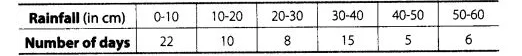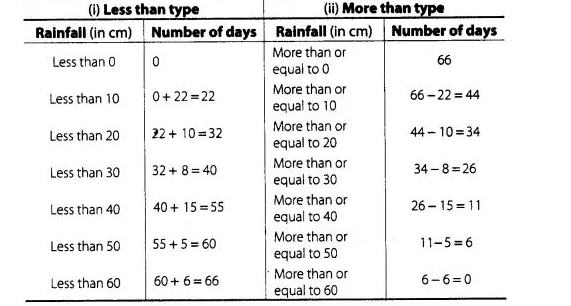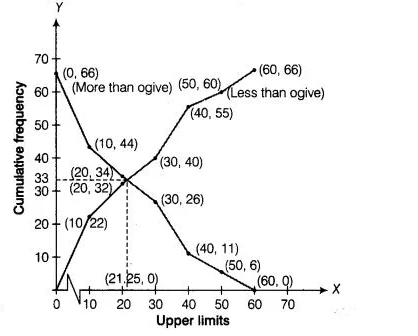# The annual rainful record of a city

Question:

The annual rainful record of a city for 66 days is given in the following table.Calculate the median rainfall using ogives (or move than type and of less than type)

Solution:

We observe that, the annual rainfall record of a city less than 0 is 0. Similarly, less than 10 include the annual rainfall record of a city from 0 as well

as the annual rainfall record of a city from 0-10.v

So, the total annual rainfall record of a city for less than 10 cm is 0+ 22 =22 days. Continuing in this manner, we will get remaining less than 20,

30, 40, 50, and 60.

Also, we observe that annual rainfall record of a city for 66 days is more than or equal to 0 cm. Since, 22

days lies in the interval 0-10. So, annual rainfall record for 66-22 = 44days is more than or equal to 10 cm.

Continuing in this manner we will get remaining more than or equal to 20, 30, 40, 50 and 60.

Now, we construct a table for less than and more than type.To draw less than type ogive we plot the points (0, 0), (10, 22), (20, 32), (30, 40), (40, 55), (50, 60), (60, 66) on the paper and join them by free

hand.

To draw the more than type ogive we plot the points (0, 66), (10, 44), (20, 34), (30, 26), (40, 11), (50, 6) and (60, 0) on the graph paper and join

them by free hand,$\because$ Total number of days $(n)=66$

Now, $\frac{n}{2}=33$

Firstly, we plot a line parallel to X-axis at intersection point of both ogives, which further intersect at (0, 33) on Y-axis. Now, we draw a line

perpendicular to X-axis at intersection point of both ogives, which further intersect at (21.25, 0) on X-axis. Which is the required median using

ogives.

Hence, median rainfall = 21.25 cm.